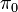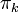# Homotopy of compact non-orientable surfaces

Jump to: navigation, search
This article describes the value (and the process used to compute it) of some homotopy invariant(s) for a topological space or family of topological spaces. The invariant is homotopy and the topological space/family is compact non-orientable surface
Get more specific information about compact non-orientable surface | Get more computations of homotopy

## Statement

This article describes the homotopy groups, including the set of path components$\pi_0$, the fundamental group$\pi_1$, and the higher homotopy groups$\pi_k$ of the compact non-orientable surface$P_n$, which is defined as the connected sum of$n$ copies of the real projective plane$\mathbb{P}^2(\R)$.

### Set of path components

Each of the spaces$P_n$ is a path-connected space, so its set of path components is a one-point space.

### Fundamental group

Fill this in later